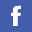Prof SHENG, Qin 盛秦教授
Institute of Creativity Distinguished Visitor
PhD, University of Cambridge; MSc, Nanjing University; BSc, Nanjing University
FSC 1107
(852) 3411-7335
ORCID

## Current Research Interests

Numerical analysis, especially numerical solution of differential equations, operator splitting, adaptation, string theory, computational physical preservations

## Selected Publications

• T. N. Jones and Q. Sheng, Asymptotic stability of a dual-scale compact method for approximating highly oscillatory Helmholtz solutions, J. Comp. Phys, 392 (2019), 403-418.
• B. Li, T. Zhu, A. Wang, K. Kirsten, G. Cleaver and Q. Sheng, Preinationary perturbations from the closed algebra approach in loop quantum cosmology, Phys. Rev. D, 99 (2019), 103536.
• L. Zhu and Q. Sheng, A note on the adaptive numerical solution of a Riemann-Liouville spacefractional Kawarada problem, J. Comput. Appl. Math., 374 (2020), 466-478.
• V. K. Singh and Q. Sheng, Bridging the science and technology by modern mathematical methods and high performance computing, Appl. Mathematics, 66 (2021), 177-181.
• Q. Sheng, J. Zhang, H.-W. Sun and J. Jin, A spatially sixth-order hybrid \$L1\$-CCD method for solving time fractional Schr.dinger equations, Appl. Mathematics, 66 (2021), 213-232.
• C. S. Chien, Yu-Tuan Lin, Q. Sheng and Yintzer Shih, A note on stochastic polynomial chaos expansions for uncertain volatility and Asian option pricing, Appl. Math. Comp., 393 (2021),
• N. Garcia-Montoya, J. Kabre, J. E. Macias-Diaz and Q. Sheng, Second-order semi-discretized schemes for solving stochastic quenching models on arbitrary spatial grids, Discrete Dynamics in Nature and Society, 21 (2021).
• T. N. Jones, E. Kostadinova, J. L. Padgett and Q. Sheng, A series representation of discrete fractional Laplace operators of arbitrary orders, 504 (2021), J. Math. Anal. Appl.
• T. N. Jones, J. L. Padgett and Q. Sheng, Intrinsic properties of strongly continuous fractional semigroups in normed vector spaces, Baylor Analysis Fest-From Operator Theory to Orthogonal Polynomials, Combinatorics, and Number Theory, Birkhauser Series in Operator Theory: Advances and Applications, Birkhauser, Berlin, Germany, 2021.
• Q. Sheng, J. E. Macias-Diaz and R. Martinez, An implicit semi-linear discretization of a bifractional Klein-Gordon-Zakharov system which conserves the total energy, Appl. Numer. Math., 169 (2021), 179-200.
• J. Kabre and Q. Sheng, A preservative splitting approximation of the solution of a variable coefficient quenching problem, Computers & Math. Appl., 100 (2021), 62-73.
• Q. Sheng, R. Martinez and J. E. Macias-Diaz, Two energy-preserving numerical models for a multi-fractional extension of the Klein-Gordon-Zakharov system, J. Comput. Appl. Math., 406 (2022), 114023, https://doi.org/10.1016/j.cam.2021.114023
• Q. Sheng, B. Villegas-Villalpando and J.E. Macias-Diaz, Real valued function spaces for which integrable functions are bounded, Mathematics, 10 (2022), 1936-1942.
• Q. Sheng and N. Garcia-Montoya, An operator splitting approach for two-dimensional Kawarada problems, Diff. Eqn. Appl. 14 (2022), 247-263.
• Q. Sheng, J. E. Macias-Diaz and R. Martinez, A nonlinear discrete model for approximating a conservative multi-fractional Zakharov system: analysis and computational simulations, Math. Computers Simulation, 202 (2022), 1-21.
• L. Zhu, N. Liu and Q. Sheng, A simulation expressivity of the quenching phenomenon in a two-sided space-fractional diffusion equations, Appl. Math. Comput., 437 (2023), 127523.
• Q. Sheng and E. S. Torres, A nonconventional stability approach for a nonlinear Crank-Nicolson method solving degenerate Kawarada problems, Appl. Math. Lett., 144 (2023), 108730.
• E. S. Torres and Q. Sheng, An accurate and preservative quenching data stream simulation method, Comm. Computer Info. Sci., (2023), to appear.